The GCHART Procedure

# Concepts

The GCHART procedure produces charts based on the values of a chart variable. These values are represented by a set of midpoints. The chart itself displays information about the chart variable in the form of chart statistics.

Terms Used with Bar Charts and Terms Used with Pie and Donut Charts illustrate these terms as well as other terms used with the GCHART procedure.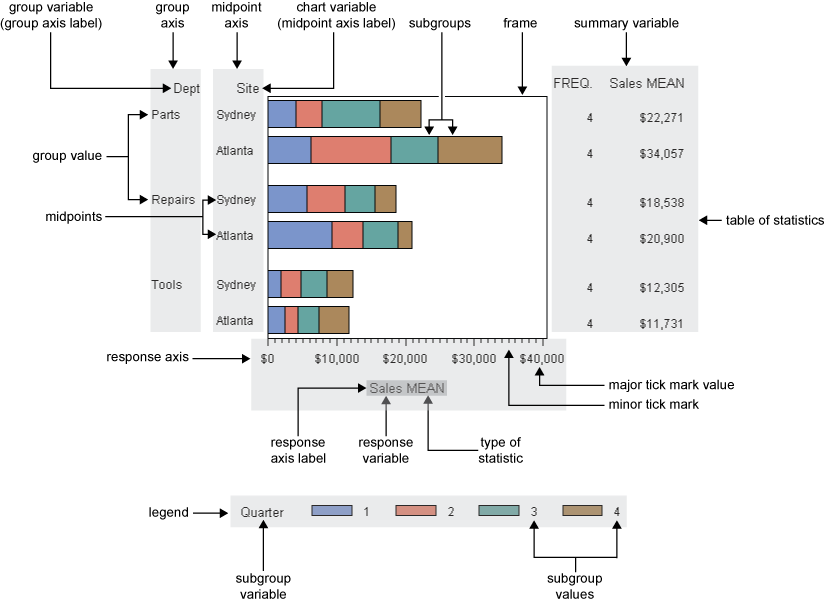Bar charts have at least two axes: a midpoint axis that shows the categories of data, and a response axis that displays the scale of values for the chart statistic. By default, the response axis is divided into evenly spaced intervals identified with major tick marks that are labeled with the corresponding statistic value. Minor tick marks are evenly distributed between the major tick marks. Each axis is labeled with the chart variable name or label. The response axis is also labeled with the statistic type.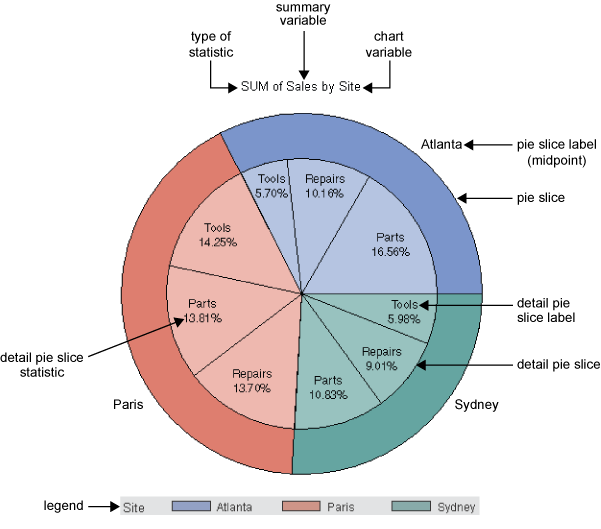Pie charts show statistics based on values of a variable called the chart variable. Generally, the values of the chart variable are represented by the slices in the chart. Beside each pie slice a number (or character string) appears that identifies the value or range of values assigned to that slice by the GCHART procedure. This number (or character string) is known as the midpoint for that slice. The statistic value for each midpoint is displayed beneath the midpoint. Each pie slice represents a different value of a given variable (the chart variable). Because the pie chart forms a circle of 360 degrees, each slice represents a percentage of degrees of the circle. The number of degrees created by each slice represents the statistic value for the midpoint.

The chart variable is the variable in the input data set whose values determine the categories of data represented by the bars, blocks, slices, or spines. The chart variable generates the midpoints to which each observation in the data set contribute.

The chart variable can be either character or numeric. Character chart variables contain character values, which are always discrete. Numeric chart variables fall into two categories: discrete and continuous.

Note:   If you apply a format that converts multiple values or a range of values to a single formatted value, then the GCHART procedure produces a single midpoint for that single formatted value.• Discrete variables contain a finite number of specific numeric values that are to be represented on the chart. For example, a variable that contains years, such as 1984 or 2001, is a discrete variable.

• Continuous variables contain a range of numeric values that are to be represented on the chart. For example, a variable of temperature data that contains real values between 0 and 212 is a continuous variable.

Numeric chart variables are always treated as continuous variables unless the DISCRETE option is used in the action statement, or, unless a format is used to group ranges of values. In most cases it is a good idea to specify the DISCRETE option when using date values.

### Missing Values

By default, the GCHART procedure ignores missing midpoint values for the chart variable. If you specify the MISSING option, then missing values are treated as a valid midpoint and are included on the chart. Missing values for the group and subgroup variables are always treated as valid groups and subgroups.

When the value of the variable that is specified in the FREQ= option is missing, 0, or negative, the observation is excluded from the calculation of the chart statistic.

When the value of the variable specified in the SUMVAR= option is missing, the observation is excluded from the calculation of the chart statistic.

Midpoints are the values of the chart variable that identify categories of data. By default, midpoints are selected or calculated by the procedure. The way the procedure handles the midpoints depends on whether the values of the chart variable are character, discrete numeric, or continuous numeric.

### Character Values

A character chart variable generates a midpoint for each unique value of the variable. For example, if the chart variable CITY contains the names of three different cities, each city is a midpoint, resulting in three midpoints for the chart: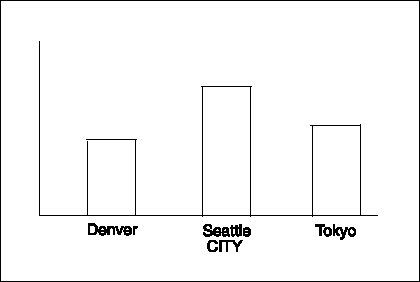(In pie charts, midpoint values that compose a small percentage of the total for the chart might be placed in the OTHER slice and will not produce a separate midpoint.)

By default, character midpoints are arranged in alphabetic order. If a character variable has an associated format, the values are arranged in order of the formatted values.

### Discrete Numeric Values

A numeric chart variable used with the DISCRETE option generates a midpoint for each unique value of the chart variable. For example, the numeric variable YEAR used with the DISCRETE option produces one midpoint for each year: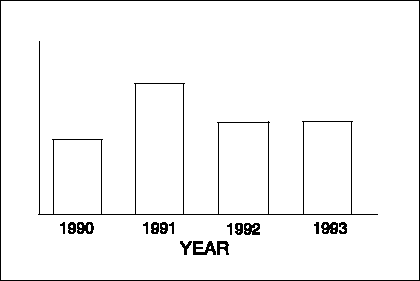By default, numeric midpoints are arranged in ascending order. The DISCRETE option is very useful for working with dates and numeric values with text user-defined formats. If the numeric variable has an associated format, each formatted value generates a separate midpoint. Formatted numeric variables are arranged in ascending order according to their unformatted numeric values.

### Continuous Numeric Values

A continuous numeric variable generates midpoints that represent ranges of values. By default, the GCHART procedure determines the ranges, calculates the median value of each range, and displays the appropriate median value at each midpoint on the chart. A value that falls exactly halfway between two midpoints is placed in the higher range.

For example, the numeric variable AGE produces four midpoints, each of which represents a ten-year age range; the median value of the range is displayed at each midpoint: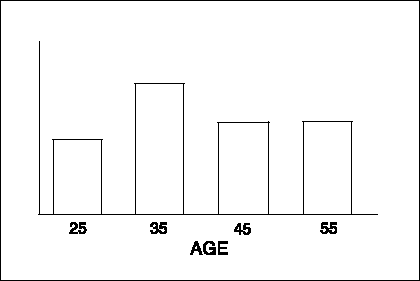By default, midpoints of ranges are arranged in ascending order.

### Selecting and Ordering Midpoints

For character or discrete numeric values, you can use the MIDPOINTS= option to rearrange the midpoints or to exclude midpoints from the chart. For example, to change the default alphabetic order of the midpoints in Character Midpoints, specify the following:

`midpoints="Tokyo" "Denver" "Seattle"`

To exclude the midpoint for Denver, specify the following:

`midpoints="Tokyo" "Seattle"`

In this case, values excluded by the option are not included in the calculation of the chart statistic.

You can order or select discrete numeric midpoint values just as you do character values, but you omit the quotation marks when specifying numeric values.

For continuous numeric variables, use the LEVELS= or MIDPOINTS= option to change the number of midpoints, to control the range of values each midpoint represents, or to change the order of the midpoints. To control the range of values each midpoint represents, use the MIDPOINTS= option to specify the median value of each range. For example, to select the ranges 20-29, 30-39, and 40-49, specify the following:

`midpoints=25 35 45`

Alternatively, to select the number of midpoints that you want and let the procedure calculate the ranges and medians, use the LEVELS= option.

You can also use formats to control the ranges of continuous numeric variables, but in that case the values are no longer continuous but discrete.

Note:   You cannot use the MIDPOINTS= option to exclude continuous numeric values from the chart. Values below or above the ranges specified by the option are automatically included in the first and last midpoints, respectively. To exclude continuous numeric values from a chart, use a WHERE statement in a DATA step or the WHERE= DATA set option.See also the description of the LEVELS= and MIDPOINTS= options for the appropriate statement.

The chart statistic is the statistical value calculated for the chart variable and represented by each block, bar, or slice. The GCHART procedure calculates six chart statistics; the default statistic is frequency.

The examples given in the descriptions of these statistics assume a data set with two variables, CITY and SALES. The values of CITY are Denver, Seattle, and Tokyo. There are 21 observations: seven for Denver, nine for Seattle, and five for Tokyo.

### Frequency

The frequency statistic is the total number of observations in the data set for each midpoint. For example, seven observations of the chart variable, CITY, contain the value Denver, so the frequency for the Denver midpoint is 7.

### Cumulative Frequency

The cumulative frequency statistic adds the frequency for the current midpoint to the frequency of all of the preceding midpoints. For example, the frequency for the Denver midpoint is 7, and the frequency for the next midpoint, Seattle, is 9, so the cumulative frequency for Seattle is 16.

You cannot request cumulative frequency with the DONUT, PIE, PIE3D, or STAR statements.

### Percentage

The percentage statistic is calculated by dividing the frequency for each midpoint by the total frequency count for all midpoints in the chart or group and multiplying it by 100. For example, the frequency count for the Denver midpoint is 7 and the total frequency count for the chart is 21, so the percentage statistic for Denver is 33.3%.

### Cumulative Percentage

The cumulative percentage statistic adds the percentage for the current midpoint to the percentage for all of the preceding midpoints in the chart or group. For example, the percentage for the Denver midpoint is 33.3, and the percentage for the next midpoint, Seattle, is 42.9, so the cumulative percentage for Seattle is 76.2.

You cannot request cumulative percentage with the DONUT, PIE, PIE3D, or STAR statements.

### Sum

The sum statistic is the total of the values for the SUMVAR= variable for each midpoint. For example, if you specify SUMVAR=SALES, and the values of the SALES variable for the seven Denver observations are 8734, 982, 1504, 3207, 4502, 624, and 918, then the sum statistic for the Denver midpoint is 20,471.

You must use the SUMVAR= option to specify the variable for which you want the sum statistic.

### Mean

The mean statistic is the average of the values for the SUMVAR= variable for each midpoint. For example, if TYPE=MEAN and SUMVAR=SALES, the mean statistic for the Denver midpoint is 2924.42.

You must use the SUMVAR= option to specify the variable for which you want the mean statistic.

### Calculating Weighted Statistics

By default, each observation is counted only once in the calculation of the chart statistic. To calculate weighted statistics in which an observation can be counted more than once, use the FREQ= option. This option identifies a variable whose values are used as a multiplier for the observation in the calculation of the statistic. If the value of the FREQ= variable is missing, 0, or negative, the observation is excluded from the calculation.

If you use the SUMVAR= option, then the SUMVAR= variable value for an observation is multiplied by the FREQ= variable value for that observation when calculating the chart statistic.

For example, to use a variable called COUNT to produce weighted statistics, assign FREQ=COUNT. If you also assign the variable HEIGHT to the SUMVAR= option, then the following table shows how the values of COUNT and HEIGHT would affect the statistic calculation:

Value of COUNT Value of HEIGHT Number of times the observation is used Value used for HEIGHT
1 55 1 55
5 65 5 325
. 63 0 -
-3 60 0 -

By default, the percentage and cumulative percentage statistics are calculated based on the frequency. If you want to chart a percentage or cumulative percentage based on a sum, you can use the FREQ= option to specify a variable to use for the "sum" calculation and specify the PCT statistic, as shown in this example:

`freq=count type=pct`

Because the variable that is used by the FREQ= option determines the number of times an observation is counted, the value of COUNT is the equivalent of the sum statistic.

See also the descriptions of the TYPE=, SUMVAR=, and FREQ= options for the action statements.

When a chart needs one or more patterns, the procedure uses either one of the following:

• default patterns and outlines that are automatically generated by SAS/GRAPH

• patterns, colors, outlines, and images that are defined by PATTERN statements, graphics options, and procedure options

The following sections summarize pattern behavior for the GCHART procedure. For more information, see PATTERN Statement.

### Default Patterns and Outlines

The GCHART procedure uses default patterns and outlines when you do not do the following:

• specify any PATTERN statements

• use the CPATTERN= graphics option

• use the COLORS= graphics options

• use the COUTLINE= option in the action statement

The default patterns, colors, and outlines are generated from the current style. If all of the above conditions are true, and the GSTYLE option is in effect, then the GCHART procedure does the following:
• selects the default fill, which is always solid, and rotates it through the color list of the current style, generating one solid pattern for each color. If the first color in the style's color list is black (or white), the procedure skips that color and begins generating patterns with the next color.

• uses the style outline color to outline every patterned area.

If all of the above conditions are true, and the NOGSTYLE option is specified then the GCHART procedure does the following:
• selects the first default fill, which is always solid, and rotates it through the device's color list, generating one solid pattern for each color. If the first color in the device's color list is black (or white), the procedure skips that color and begins generating patterns with the next color.

• uses the foreground color to outline every patterned area.

• if the procedure needs additional patterns, GCHART selects the next default pattern fill that is appropriate to the type of chart and rotates it through the color on the list, skipping the foreground color as before. The procedure continues in this manner until it has generated enough patterns for the chart.

Changing any of the above conditions changes or overrides the default behavior:

• If you specify a color list with the COLORS= option in a GOPTIONS statement and the list contains more than one color, the procedure produces a solid pattern through that list, using every color, even if the foreground color is black (or white). The default outline color remains the style outline color.

• If you specify either COLORS=(one-color) or the CPATTERN= graphics option, the default fill pattern changes from solid to the list of appropriate hatch patterns. The procedure uses the specified color to generate one pattern definition for each hatch pattern in the list. The default outline color remains the style outline color. (The Java and ActiveX devices do not support hatch patterns.)

For a description of these graphics options, see Graphics Options and Device Parameters Dictionary.

### User-Defined Patterns, Outlines, and Images

You can use PATTERN statements to specify patterns, including color or fill type or both. You can also specify images to fill the bars of two-dimensional bar charts. For complete information on all patterns, see PATTERN Statement. See also the section on controlling patterns and colors for each chart type.

When you use PATTERN statements, the procedure uses the specified patterns until all of the PATTERN definitions they generate have been used. Then, if more patterns are required, it returns to the default pattern rotation.

To change the outline color of any pattern, whether it's a default or user-defined pattern, use the COUTLINE= option in the action statement that generates the chart.

Two-dimensional bar charts created with the HBAR and VBAR statements can use the PATTERN statement to fill specified bars with specified images. For details, see the IMAGE= option. Other means of including images in charts include adding background images to bar charts. The IBACK= option specifies an image file that fills the entire area behind the graph. The IFRAME= option specifies an image file that fills the area within the axes of the graph.

For additional information, including a listing of recognized image file types, see Image File Types Supported by SAS/GRAPH.Previous Page | Next Page | Top of Page